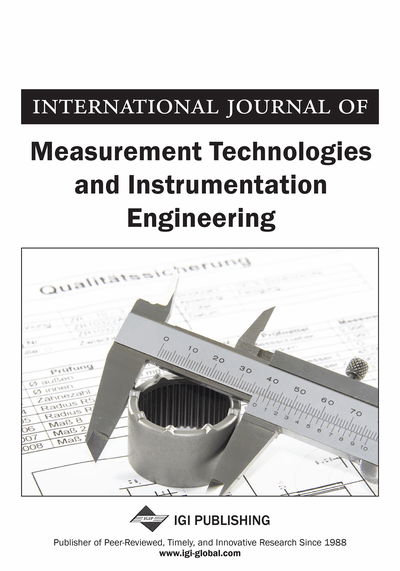# Revenue Efficiency of Fuzzy Sample Decision Making Unit

Nazila Aghayi (Department of Mathematics, Ardabil Brach, Islamic Azad University, Ardabil, Iran) and Samira Salehpour (Young Researchers and Elite Club, Ardabil Branch, Islamic Azad University, Ardabil, Iran)
DOI: 10.4018/IJMTIE.2015070102
Available
\$37.50
No Current Special Offers

## Abstract

Revenue efficiency measurement is one of the most important issues in data envelopment analysis (DEA). Most of the proposed models calculate the revenue efficiency of decision making units (DMUs) which play a main role in the formation of the production possibility set by implementing exact or fuzzy data. The revenue efficiency value of a sample decision making unit with exact and fuzzy data has not been investigated by these models yet. There exist different types of fuzzy numbers, however, only a special type of them has been used in revenue efficiency models with fuzzy data. The concept of vector has not been employed to calculate the measure of the revenue efficiency in any of the studies conducted thus far. However, in this article the authors propose a model for evaluating the revenue efficiency measure of a fuzzy sample DMU without the limitations of previous models with regards to the formation of the production possibility set. In the proposed model, data can be selected from different types of fuzzy numbers and there is no limitation on the type of the used fuzzy data. In addition, the current article employs the concept of vector for revenue efficiency assessment for the first time.
Article Preview
Top

## 1. Introduction

Data envelopment analysis (DEA) is a nonparametric method which utilizes linear programming (LP) technique to estimate the best production frontier (efficient), and evaluates the efficiency of a set of homogeneous units. Efficiency is defined as a weighted sum of outputs divided

by a weighted sum of inputs. Revenue efficiency is a DEA model that enables a single DMU to earn more revenues measured using current inputs. Farrell (1957) firstly introduced a method for the measurement of productive efficiency, then used the observed inputs and outputs of a set of selected firms as a standard for measuring the productivity of the firms rather than production function estimation. However, the Farrell’s model was basically to measure cost efficiency, it had the potential to be easily transformed into a revenue efficiency model via minor changes. Using data envelopment analysis, Fare et al. (1985) took a practical approach toward cost efficiency (CE) and revenue efficiency (RE). Subsequently, so many studies were done on the measurement of revenue efficiency. Toloo et al. (2008) introduced a model to measure overall profit efficiency of DMUs with interval data. Rostamy-Malkhalifeh and Aghayi (2011) proposed two Ranking methods for Units based on their overall profit efficiency with interval data. Rostamy-Malkhalifeh and Aghayi (2011) presented the new model for measuring overall profit efficiency of DMUs with fuzzy data. Based on the proposed model by Kuosmanen and Post (2001), Camanho and Dyson (2005) provided a model for the calculation of cost efficiency with imprecise input prices in both optimistic and pessimistic conditions. Emrouznejad et al. (2011) suggested an overall profit malmquist productivity index with fuzzy and interval data. Salehpour and Aghayi (2015) evaluated most revenue efficiency of DMUs with price uncertainty. Aghayi and Ghelejbeigi (2016) improved cost efficiency of DMUs by resource allocation. Mostafaee and Saljooghi (2010) used uncertain data to devise a method for calculating the upper and lower bounds of cost efficiency. Aghayi et al. (2016) suggested robust efficiency measurement with common set of weights under varying degrees of conservatism and data uncertainty. Aghayi (2016) introduced cost efficiency measurement with fuzzy data in DEA. Kuosmanen et al. (2010) performed a firm and industry level profit efficiency analysis using shadow prices. Aghayi and Maleki (2016) proposed efficiency measurement of DMUs with undesirable outputs under uncertainty based on the directional distance function. Sahoo et al. (2014) adopted distance function approach to develop models for cost, revenue, and profit efficiency measurement with price uncertainty. Their model had the potential to be used for negative data. Revenue efficiency measurement with undesirable data in fuzzy DEA is showed by Aghayi (2016).

## Complete Article List

Search this Journal:
Reset
Open Access Articles
Volume 7: 2 Issues (2018)
Volume 6: 2 Issues (2017)
Volume 5: 2 Issues (2015)
Volume 4: 2 Issues (2014)
Volume 3: 4 Issues (2013)
Volume 2: 4 Issues (2012)
Volume 1: 4 Issues (2011)
View Complete Journal Contents Listing